Скачать презентацию Franco Donzelli Topics in the History of Equilibrium

192babc31aa75b10e6db4c4c55533bfc.ppt

• Количество слайдов: 54Franco Donzelli Topics in the History of Equilibrium Analysis Lesson 3 Marshall vs. Walras on Equilibrium and Disequilibrium Ph. D. Program in Economics University of York February-March 2008 Franco Donzelli Lesson 3 - Marshall vs. Walras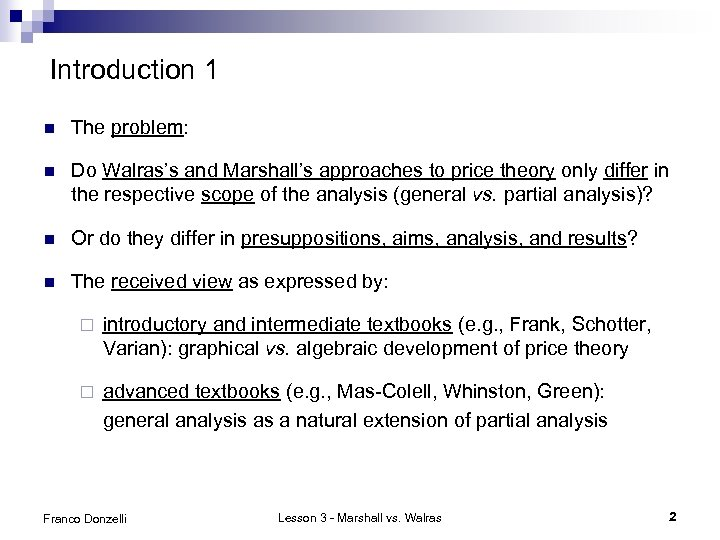Introduction 1 n The problem: n Do Walras’s and Marshall’s approaches to price theory only differ in the respective scope of the analysis (general vs. partial analysis)? n Or do they differ in presuppositions, aims, analysis, and results? n The received view as expressed by: ¨ introductory and intermediate textbooks (e. g. , Frank, Schotter, Varian): graphical vs. algebraic development of price theory ¨ advanced textbooks (e. g. , Mas-Colell, Whinston, Green): general analysis as a natural extension of partial analysis Franco Donzelli Lesson 3 - Marshall vs. Walras 2Introduction 2 n The working hypothesis: n Walras’s and Marshall’s approaches to price theory differ in essential respects. n The main differences have to do with: the basic assumptions about the functioning of the trading process ¨ the nature of competition: “perfect competition” vs. “bilateral bargaining” ¨ the nature of the disequilibrium process: in either “logical” or “real” time ¨ the interpretation of the equilibrium construct: either an “instantaneous” state or the “limit point of a sequence” in “real” time ¨ the nature of prices: numeraire normalization vs. money prices The manifest difference in the scope of the analysis, i. e. , general vs. partial analysis, is the necessary by-product of more fundamental epistemological and theoretical differences ¨ n Franco Donzelli Lesson 3 - Marshall vs. Walras 3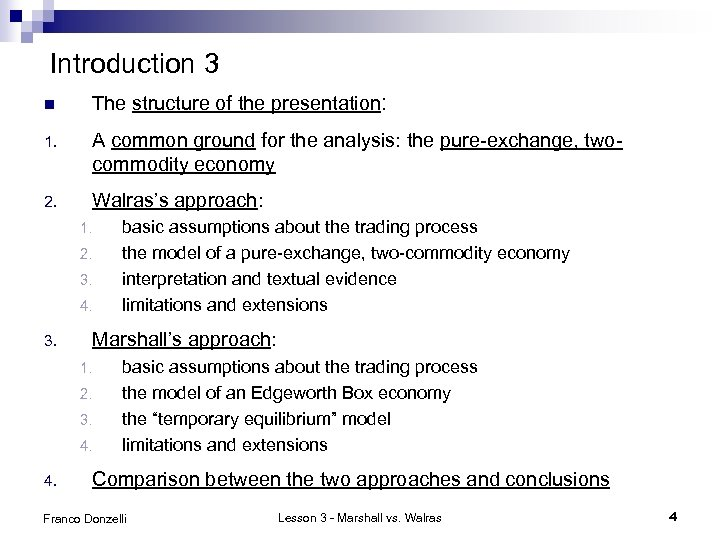Introduction 3 n The structure of the presentation: 1. A common ground for the analysis: the pure-exchange, twocommodity economy 2. Walras’s approach: 1. 2. 3. 4. 3. Marshall’s approach: 1. 2. 3. 4. basic assumptions about the trading process the model of a pure-exchange, two-commodity economy interpretation and textual evidence limitations and extensions basic assumptions about the trading process the model of an Edgeworth Box economy the “temporary equilibrium” model limitations and extensions Comparison between the two approaches and conclusions Franco Donzelli Lesson 3 - Marshall vs. Walras 4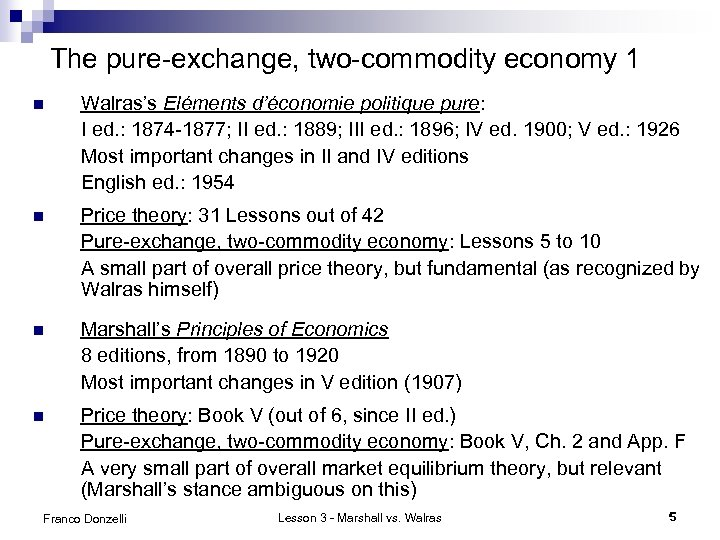The pure-exchange, two-commodity economy 1 n Walras’s Eléments d’économie politique pure: I ed. : 1874 -1877; II ed. : 1889; III ed. : 1896; IV ed. 1900; V ed. : 1926 Most important changes in II and IV editions English ed. : 1954 n Price theory: 31 Lessons out of 42 Pure-exchange, two-commodity economy: Lessons 5 to 10 A small part of overall price theory, but fundamental (as recognized by Walras himself) n Marshall’s Principles of Economics 8 editions, from 1890 to 1920 Most important changes in V edition (1907) n Price theory: Book V (out of 6, since II ed. ) Pure-exchange, two-commodity economy: Book V, Ch. 2 and App. F A very small part of overall market equilibrium theory, but relevant (Marshall’s stance ambiguous on this) Franco Donzelli Lesson 3 - Marshall vs. Walras 5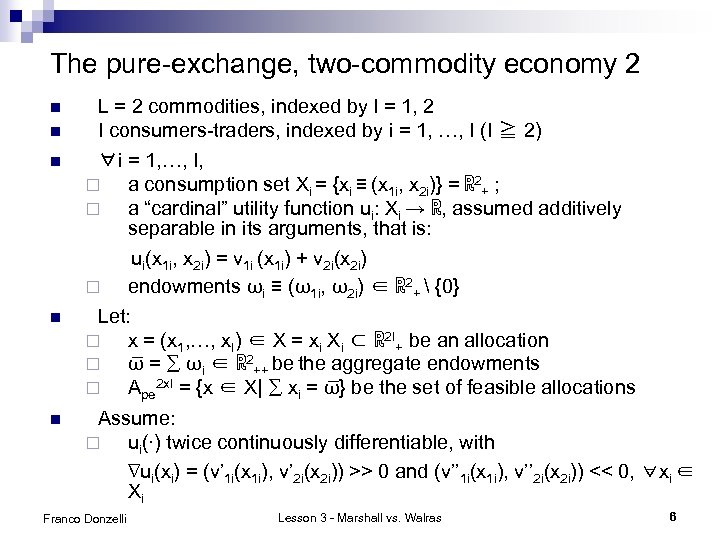The pure-exchange, two-commodity economy 2 n n n L = 2 commodities, indexed by l = 1, 2 I consumers-traders, indexed by i = 1, …, I (I ≧ 2) ∀i = 1, …, I, ¨ a consumption set Xi = {xi ≡ (x 1 i, x 2 i)} = ℝ 2+ ; ¨ a “cardinal” utility function ui: Xi → ℝ, assumed additively separable in its arguments, that is: ui(x 1 i, x 2 i) = v 1 i (x 1 i) + v 2 i(x 2 i) ¨ endowments ωi ≡ (ω1 i, ω2 i) ∈ ℝ 2+ {0} Let: ¨ x = (x 1, …, x. I) ∈ X = xi Xi ⊂ ℝ 2 I+ be an allocation ¨ ω = ∑ ωi ∈ ℝ 2++ be the aggregate endowments ¨ Ape 2 x. I = {x ∈ X| ∑ xi = ω } be the set of feasible allocations Assume: ¨ ui(∙) twice continuously differentiable, with ∇ui(xi) = (v’ 1 i(x 1 i), v’ 2 i(x 2 i)) >> 0 and (v’’ 1 i(x 1 i), v’’ 2 i(x 2 i)) << 0, ∀xi ∈ Xi Franco Donzelli Lesson 3 - Marshall vs. Walras 6The pure-exchange, two-commodity economy 3 n n n Let: ¨ ℰpe 2 x. I = {(Xi, ui(∙), ωi)Ii=1} be a pure-exchange, two-commodity economy ¨ ℰEB = ℰpe 2 x 2 = {(ℝ 2+, ui(∙), ωi)2 i=1} be an Edgeworth Box economy Given ℰpe 2 x. I, ∀x ∈ Ape 2 x. I, let MRSi 21(xi) ≡ |dx 2 i/dx 1 i|ui(xi+dxi)=u(xi) = (∂u(xi)/∂u(x 1 i) / (∂u(xi)/∂u(x 2 i) be consumer i’s marginal rate of substitution of commodity 2 for commodity 1 when his consumption is xi Let zi(xi) ≡ (z 1 i, z 2 i)(xi) ≡ xi - ωi ≡ (x 1 i - ω1 i, x 2 i - ω2 i) ∈ ℝ 2 be consumer i’s excess demand, when his consumption is xi If zli(xi) > 0, then zli(xi) is called consumer i’s net demand proper for commodity I and consumer i is said to be a net buyer If zli(xi) < 0, then |zli(xi)| is called consumer i’s net supply for commodity I and consumer i is said to be a net seller Franco Donzelli Lesson 3 - Marshall vs. Walras 7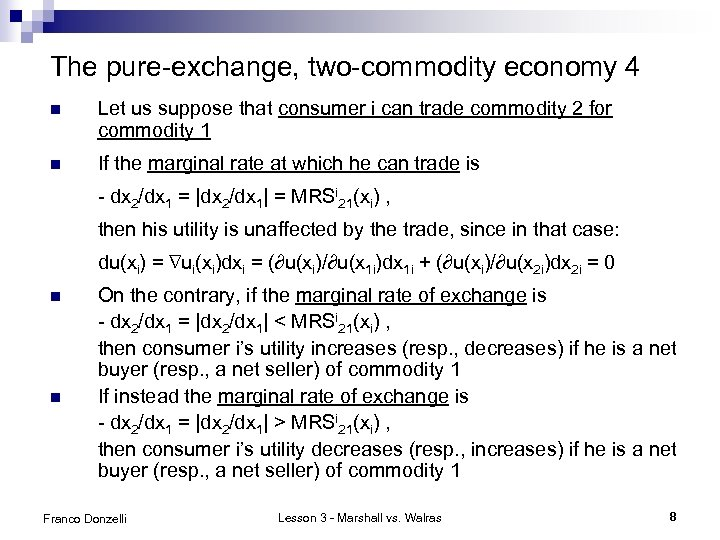The pure-exchange, two-commodity economy 4 n Let us suppose that consumer i can trade commodity 2 for commodity 1 n If the marginal rate at which he can trade is - dx 2/dx 1 = |dx 2/dx 1| = MRSi 21(xi) , then his utility is unaffected by the trade, since in that case: du(xi) = ∇ui(xi)dxi = (∂u(xi)/∂u(x 1 i)dx 1 i + (∂u(xi)/∂u(x 2 i)dx 2 i = 0 n n On the contrary, if the marginal rate of exchange is - dx 2/dx 1 = |dx 2/dx 1| < MRSi 21(xi) , then consumer i’s utility increases (resp. , decreases) if he is a net buyer (resp. , a net seller) of commodity 1 If instead the marginal rate of exchange is - dx 2/dx 1 = |dx 2/dx 1| > MRSi 21(xi) , then consumer i’s utility decreases (resp. , increases) if he is a net buyer (resp. , a net seller) of commodity 1 Franco Donzelli Lesson 3 - Marshall vs. Walras 8The pure-exchange, two-commodity economy 5 n Hence the marginal rate of substitution of commodity 2 for commodity 1, MRSi 21(xi), can also be interpreted as the maximum (resp. , minimum) quantity of commodity 2 that a utility maximizing buyer (resp. , seller) of commodity 1 is willing to pay (resp. , to receive) at the margin in exchange for one unit of commodity 1, when his consumption is xi. n MRSi 21(xi) represents consumer i’s “reservation price” of commodity 1 in terms of commodity 2, when his consumption is xi. n Both Walras and Marshall do not exactly employ the above conceptual apparatus n They do not make any strong monotonicity assumption, ∇ui(xi) = (v’ 1 i(x 1 i), v’ 2 i(x 2 i)) >> 0; Walras explicitly allows for consumers to become satiated at finite consumption bundles. But to assume non-satiation is an unobtrusive simplifying assumption. n They both ignore both the notion of marginal rate of substitution and that of reservation price. Franco Donzelli Lesson 3 - Marshall vs. Walras 9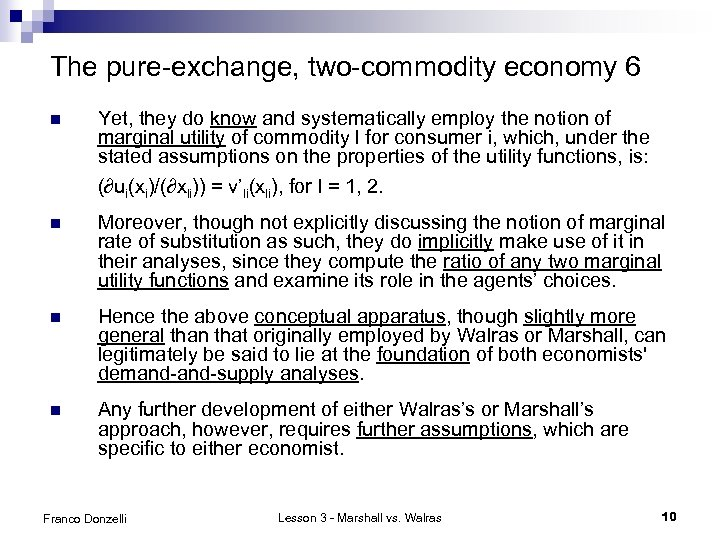The pure-exchange, two-commodity economy 6 n Yet, they do know and systematically employ the notion of marginal utility of commodity l for consumer i, which, under the stated assumptions on the properties of the utility functions, is: (∂ui(xi)/(∂xli)) = v’li(xli), for l = 1, 2. n Moreover, though not explicitly discussing the notion of marginal rate of substitution as such, they do implicitly make use of it in their analyses, since they compute the ratio of any two marginal utility functions and examine its role in the agents’ choices. n Hence the above conceptual apparatus, though slightly more general than that originally employed by Walras or Marshall, can legitimately be said to lie at the foundation of both economists' demand-supply analyses. n Any further development of either Walras’s or Marshall’s approach, however, requires further assumptions, which are specific to either economist. Franco Donzelli Lesson 3 - Marshall vs. Walras 10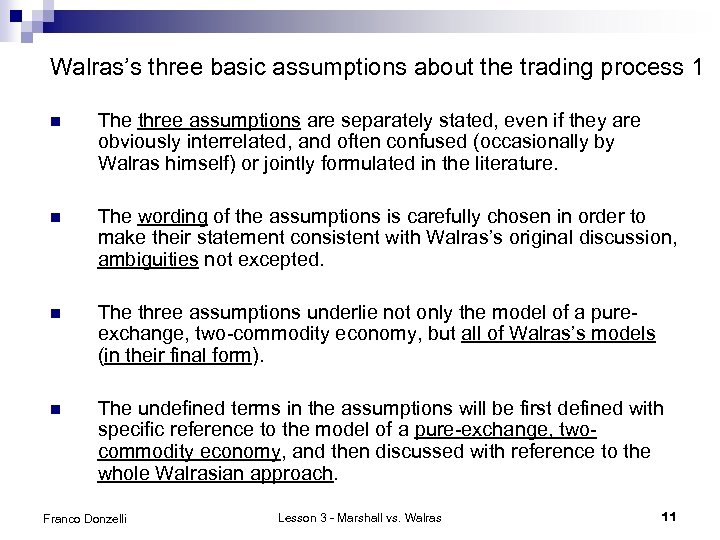Walras’s three basic assumptions about the trading process 1 n The three assumptions are separately stated, even if they are obviously interrelated, and often confused (occasionally by Walras himself) or jointly formulated in the literature. n The wording of the assumptions is carefully chosen in order to make their statement consistent with Walras’s original discussion, ambiguities not excepted. n The three assumptions underlie not only the model of a pureexchange, two-commodity economy, but all of Walras’s models (in their final form). n The undefined terms in the assumptions will be first defined with specific reference to the model of a pure-exchange, twocommodity economy, and then discussed with reference to the whole Walrasian approach. Franco Donzelli Lesson 3 - Marshall vs. Walras 11Walras’s three basic assumptions about the trading process 2 n Assumption 1. (“Law of one price" or "Jevons' law of indifference") At each instant of the trading process, a price is quoted in the market for each commodity. Moreover, if any transaction concerning a given commodity takes place at any instant of the trading process, then it takes place at the price quoted at that instant. n Assumption 2. ("Perfect competition") All traders behave competitively, that is, they take prices as given parameters in making their optimizing choices. n Assumption 3. ("No trade out of equilibrium") No transaction concerning any commodity is allowed to take place out of equilibrium. Franco Donzelli Lesson 3 - Marshall vs. Walras 12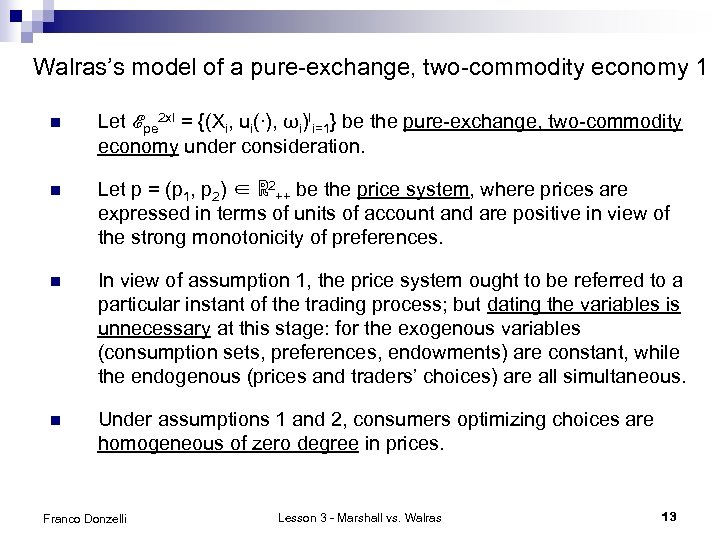Walras’s model of a pure-exchange, two-commodity economy 1 n Let ℰpe 2 x. I = {(Xi, ui(∙), ωi)Ii=1} be the pure-exchange, two-commodity economy under consideration. n Let p = (p 1, p 2) ∈ ℝ 2++ be the price system, where prices are expressed in terms of units of account and are positive in view of the strong monotonicity of preferences. n In view of assumption 1, the price system ought to be referred to a particular instant of the trading process; but dating the variables is unnecessary at this stage: for the exogenous variables (consumption sets, preferences, endowments) are constant, while the endogenous (prices and traders’ choices) are all simultaneous. n Under assumptions 1 and 2, consumers optimizing choices are homogeneous of zero degree in prices. Franco Donzelli Lesson 3 - Marshall vs. Walras 13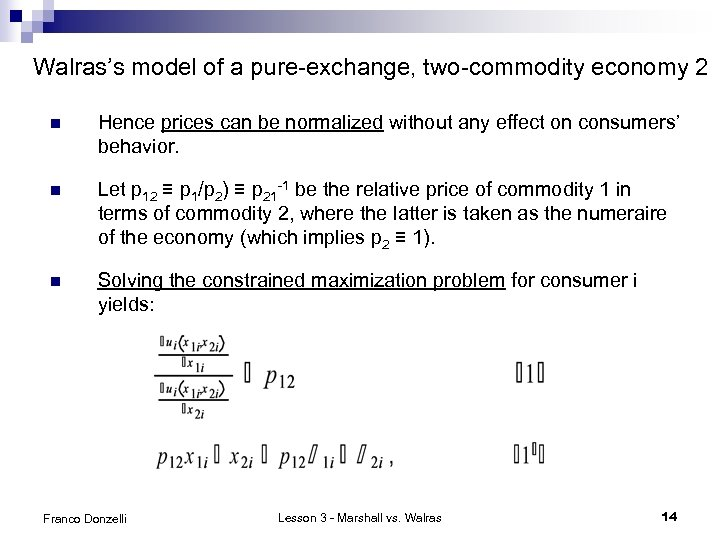Walras’s model of a pure-exchange, two-commodity economy 2 n Hence prices can be normalized without any effect on consumers’ behavior. n Let p 12 ≡ p 1/p 2) ≡ p 21 -1 be the relative price of commodity 1 in terms of commodity 2, where the latter is taken as the numeraire of the economy (which implies p 2 ≡ 1). n Solving the constrained maximization problem for consumer i yields: Franco Donzelli Lesson 3 - Marshall vs. Walras 14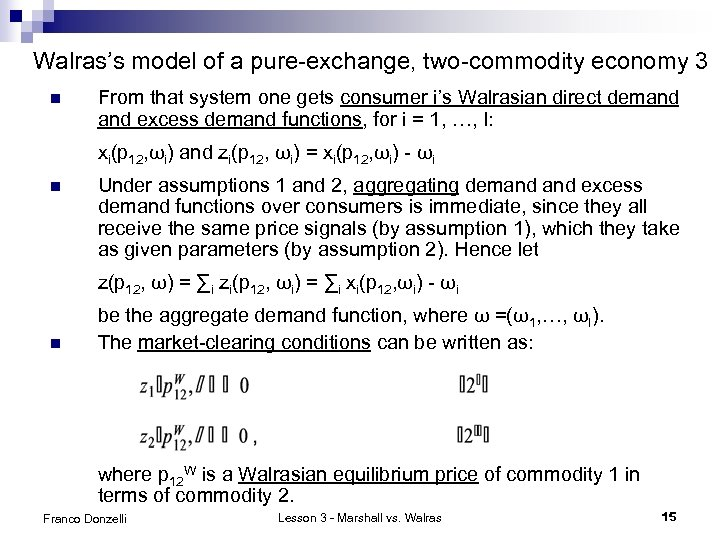Walras’s model of a pure-exchange, two-commodity economy 3 n From that system one gets consumer i’s Walrasian direct demand excess demand functions, for i = 1, …, I: xi(p 12, ωi) and zi(p 12, ωi) = xi(p 12, ωi) - ωi n Under assumptions 1 and 2, aggregating demand excess demand functions over consumers is immediate, since they all receive the same price signals (by assumption 1), which they take as given parameters (by assumption 2). Hence let z(p 12, ω) = ∑i zi(p 12, ωi) = ∑i xi(p 12, ωi) - ωi n be the aggregate demand function, where ω =(ω1, …, ωI). The market-clearing conditions can be written as: where p 12 W is a Walrasian equilibrium price of commodity 1 in terms of commodity 2. Franco Donzelli Lesson 3 - Marshall vs. Walras 15Walras’s model of a pure-exchange, two-commodity economy 4 n From budget equations, by rearranging terms and summing over consumers, we get the so-called Walras’ Law: n Due to Walras’ Law, equation (2’’) is necessarily satisfied when equation (2’) holds. Hence we can focus on equation (2’). n Equation (2’) has at least one solution, not necessarily unique under the stated assumptions. n Each solution yields a Walrasian equilibrium price of commodity 1 in terms of commodity 2, p 12 W, to which a Walrasian equilibrium allocation x(p 12 W) = (x 1(p 12 W), …, xi(p 12 W), …, x. I(p 12 W)) is associated. Franco Donzelli Lesson 3 - Marshall vs. Walras 16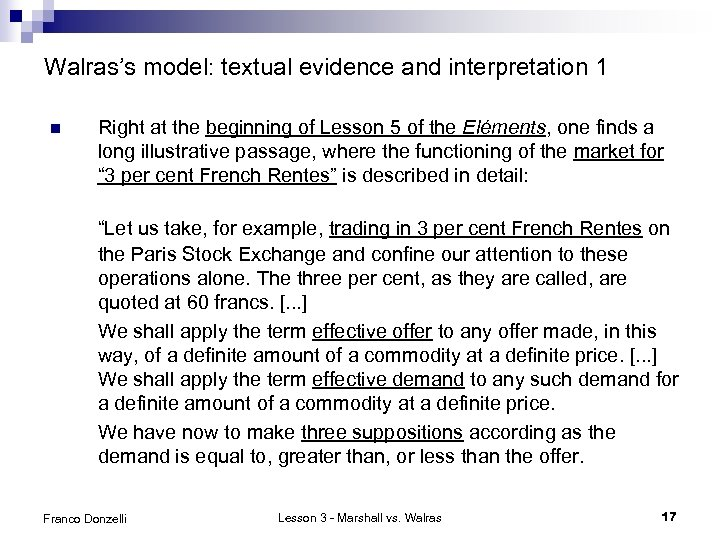Walras’s model: textual evidence and interpretation 1 n Right at the beginning of Lesson 5 of the Eléments, one finds a long illustrative passage, where the functioning of the market for “ 3 per cent French Rentes” is described in detail: “Let us take, for example, trading in 3 per cent French Rentes on the Paris Stock Exchange and confine our attention to these operations alone. The three per cent, as they are called, are quoted at 60 francs. [. . . ] We shall apply the term effective offer to any offer made, in this way, of a definite amount of a commodity at a definite price. [. . . ] We shall apply the term effective demand to any such demand for a definite amount of a commodity at a definite price. We have now to make three suppositions according as the demand is equal to, greater than, or less than the offer. Franco Donzelli Lesson 3 - Marshall vs. Walras 17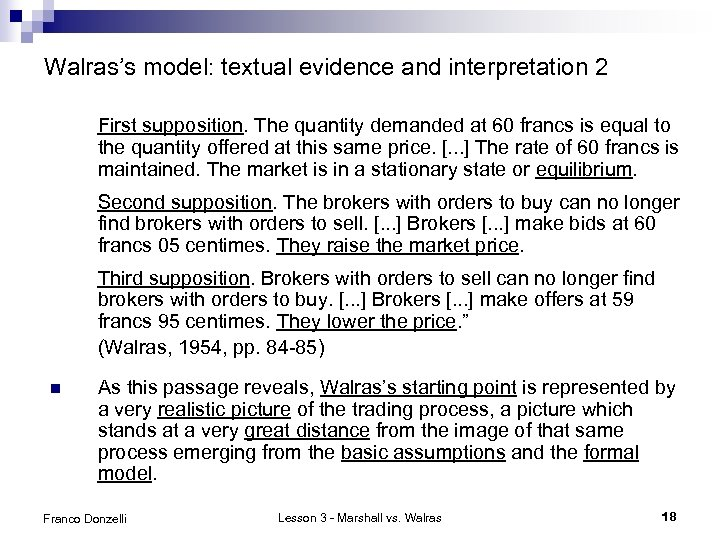Walras’s model: textual evidence and interpretation 2 First supposition. The quantity demanded at 60 francs is equal to the quantity offered at this same price. [. . . ] The rate of 60 francs is maintained. The market is in a stationary state or equilibrium. Second supposition. The brokers with orders to buy can no longer find brokers with orders to sell. [. . . ] Brokers [. . . ] make bids at 60 francs 05 centimes. They raise the market price. Third supposition. Brokers with orders to sell can no longer find brokers with orders to buy. [. . . ] Brokers [. . . ] make offers at 59 francs 95 centimes. They lower the price. ” (Walras, 1954, pp. 84 -85) n As this passage reveals, Walras’s starting point is represented by a very realistic picture of the trading process, a picture which stands at a very great distance from the image of that same process emerging from the basic assumptions and the formal model. Franco Donzelli Lesson 3 - Marshall vs. Walras 18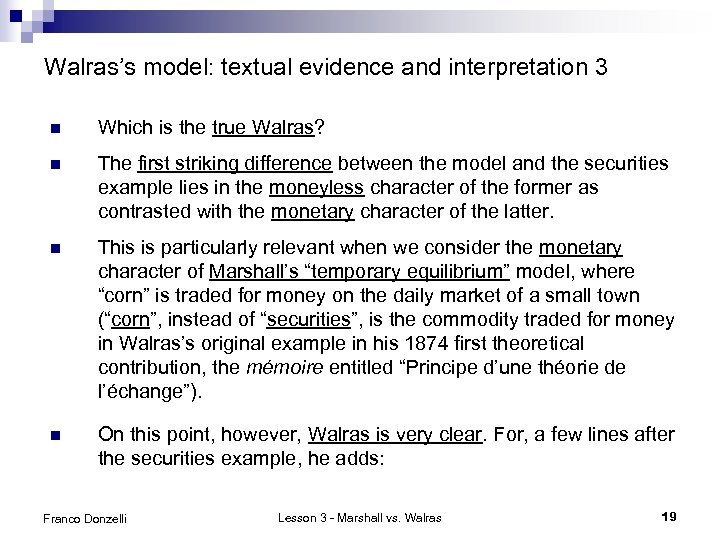Walras’s model: textual evidence and interpretation 3 n Which is the true Walras? n The first striking difference between the model and the securities example lies in the moneyless character of the former as contrasted with the monetary character of the latter. n This is particularly relevant when we consider the monetary character of Marshall’s “temporary equilibrium” model, where “corn” is traded for money on the daily market of a small town (“corn”, instead of “securities”, is the commodity traded for money in Walras’s original example in his 1874 first theoretical contribution, the mémoire entitled “Principe d’une théorie de l’échange”). n On this point, however, Walras is very clear. For, a few lines after the securities example, he adds: Franco Donzelli Lesson 3 - Marshall vs. Walras 19Walras’s model: textual evidence and interpretation 4 n “Securities, however, are a very special kind of commodity. Furthermore, the use of money in trading has peculiarities of its own, the study of which must be postponed until later, and not interwoven at the outset with the general phenomenon of value in exchange. Let us, therefore, retrace our steps and state our observations in scientific terms. We may take any two commodities, say oats and wheat, or, more abstractly, (A) and (B). ” (Walras, 1954, pp. 86 -87) n Coming now to the three basic assumptions about the trading process, we see that all three of them are apparently disconfirmed in the securities example: ¨ ¨ ¨ Franco Donzelli traders “make” prices, so that assumption 2 is violated; different price bids can apparently coexist in time, so that also assumption 1 fails; trades can actually occur at out-of-equilibrium prices, so that assumption 3 is violated as well. Lesson 3 - Marshall vs. Walras 20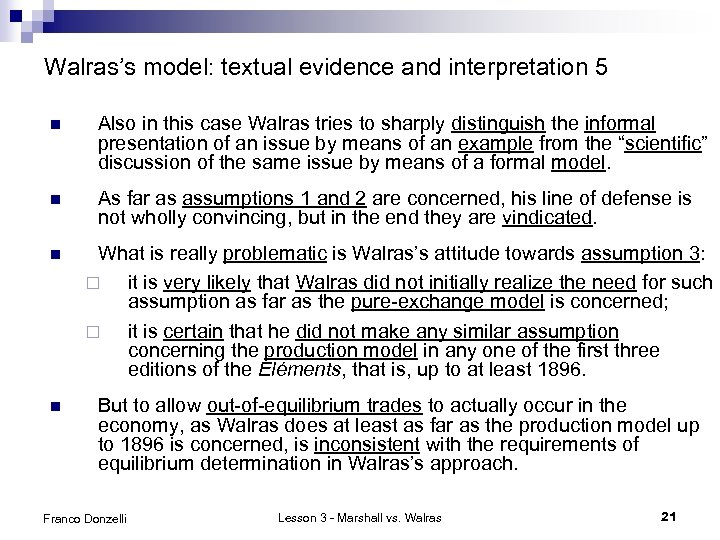Walras’s model: textual evidence and interpretation 5 n Also in this case Walras tries to sharply distinguish the informal presentation of an issue by means of an example from the “scientific” discussion of the same issue by means of a formal model. n As far as assumptions 1 and 2 are concerned, his line of defense is not wholly convincing, but in the end they are vindicated. n What is really problematic is Walras’s attitude towards assumption 3: ¨ it is very likely that Walras did not initially realize the need for such assumption as far as the pure-exchange model is concerned; ¨ it is certain that he did not make any similar assumption concerning the production model in any one of the first three editions of the Eléments, that is, up to at least 1896. n But to allow out-of-equilibrium trades to actually occur in the economy, as Walras does at least as far as the production model up to 1896 is concerned, is inconsistent with the requirements of equilibrium determination in Walras’s approach. Franco Donzelli Lesson 3 - Marshall vs. Walras 21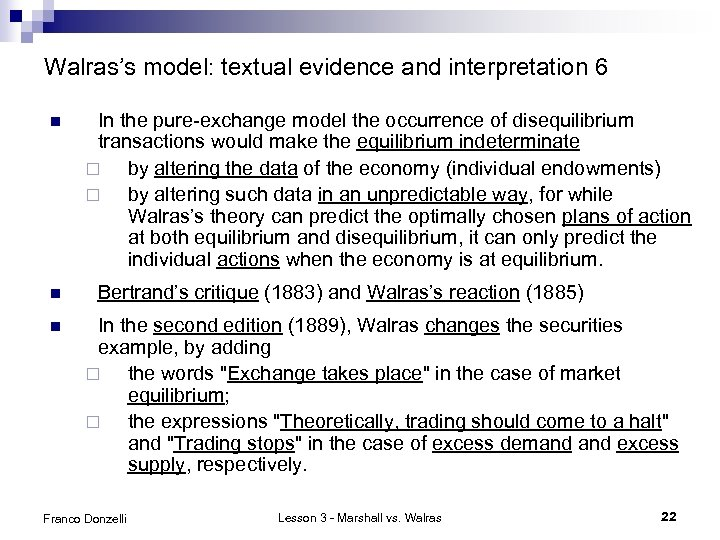Walras’s model: textual evidence and interpretation 6 n n n In the pure-exchange model the occurrence of disequilibrium transactions would make the equilibrium indeterminate ¨ by altering the data of the economy (individual endowments) ¨ by altering such data in an unpredictable way, for while Walras’s theory can predict the optimally chosen plans of action at both equilibrium and disequilibrium, it can only predict the individual actions when the economy is at equilibrium. Bertrand’s critique (1883) and Walras’s reaction (1885) In the second edition (1889), Walras changes the securities example, by adding ¨ the words "Exchange takes place" in the case of market equilibrium; ¨ the expressions "Theoretically, trading should come to a halt" and "Trading stops" in the case of excess demand excess supply, respectively. Franco Donzelli Lesson 3 - Marshall vs. Walras 22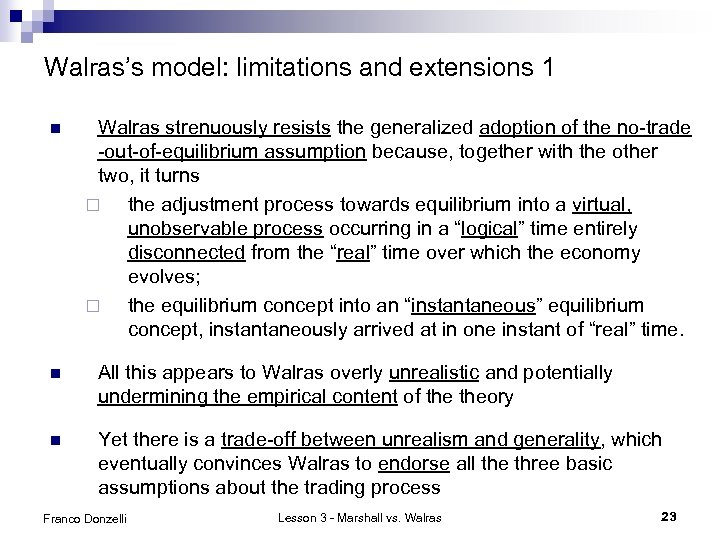Walras’s model: limitations and extensions 1 n Walras strenuously resists the generalized adoption of the no-trade -out-of-equilibrium assumption because, together with the other two, it turns ¨ the adjustment process towards equilibrium into a virtual, unobservable process occurring in a “logical” time entirely disconnected from the “real” time over which the economy evolves; ¨ the equilibrium concept into an “instantaneous” equilibrium concept, instantaneously arrived at in one instant of “real” time. n All this appears to Walras overly unrealistic and potentially undermining the empirical content of theory n Yet there is a trade-off between unrealism and generality, which eventually convinces Walras to endorse all the three basic assumptions about the trading process Franco Donzelli Lesson 3 - Marshall vs. Walras 23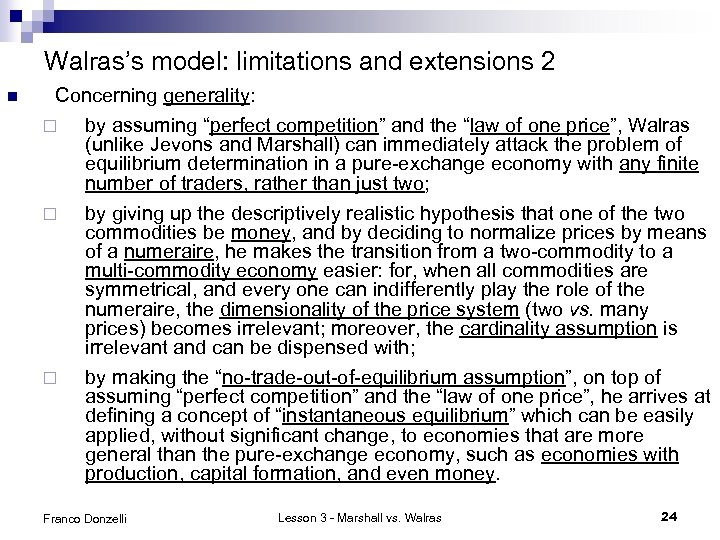Walras’s model: limitations and extensions 2 n Concerning generality: ¨ by assuming “perfect competition” and the “law of one price”, Walras (unlike Jevons and Marshall) can immediately attack the problem of equilibrium determination in a pure-exchange economy with any finite number of traders, rather than just two; ¨ ¨ by giving up the descriptively realistic hypothesis that one of the two commodities be money, and by deciding to normalize prices by means of a numeraire, he makes the transition from a two-commodity to a multi-commodity economy easier: for, when all commodities are symmetrical, and every one can indifferently play the role of the numeraire, the dimensionality of the price system (two vs. many prices) becomes irrelevant; moreover, the cardinality assumption is irrelevant and can be dispensed with; by making the “no-trade-out-of-equilibrium assumption”, on top of assuming “perfect competition” and the “law of one price”, he arrives at defining a concept of “instantaneous equilibrium” which can be easily applied, without significant change, to economies that are more general than the pure-exchange economy, such as economies with production, capital formation, and even money. Franco Donzelli Lesson 3 - Marshall vs. Walras 24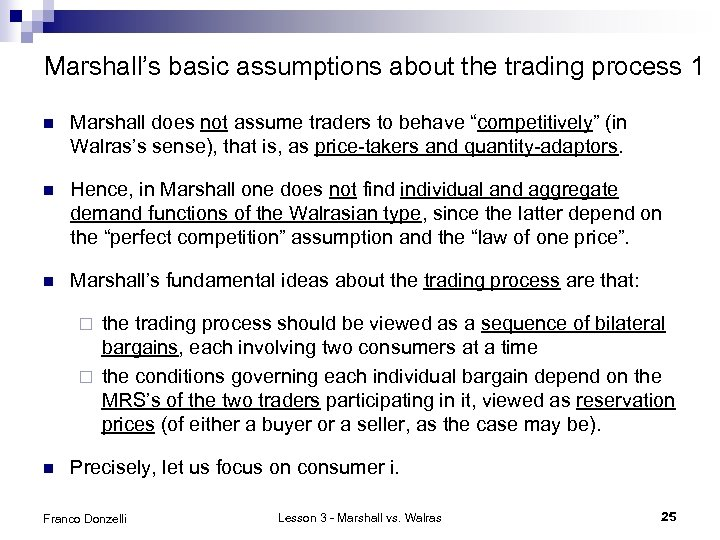Marshall’s basic assumptions about the trading process 1 n Marshall does not assume traders to behave “competitively” (in Walras’s sense), that is, as price-takers and quantity-adaptors. n Hence, in Marshall one does not find individual and aggregate demand functions of the Walrasian type, since the latter depend on the “perfect competition” assumption and the “law of one price”. n Marshall’s fundamental ideas about the trading process are that: the trading process should be viewed as a sequence of bilateral bargains, each involving two consumers at a time ¨ the conditions governing each individual bargain depend on the MRS’s of the two traders participating in it, viewed as reservation prices (of either a buyer or a seller, as the case may be). ¨ n Precisely, let us focus on consumer i. Franco Donzelli Lesson 3 - Marshall vs. Walras 25Marshall’s basic assumptions about the trading process 2 n Let MRS 21 i(ω1 i, ω2 i) = (∂u(ω1 i, ω2 i)/∂u(x 1 i) / (∂u(ω1 i, ω2 i)/∂u(x 2 i) be the initial value of consumer i’s marginal rate of substitution of commodity 2 for commodity 1 n Supposing ∃j s. t. j ≠ i and MRS 21 j(ω1 j, ω2 j) ≠ MRS 21 i(ω1 i, ω2 i), let kij(ω) = min {MRS 21 i(ω1 i, ω2 i), MRS 21 j(ω1 j, ω2 j)} and Kij(ω) = max {MRS 21 i(ω1 i, ω2 i), MRS 21 j(ω1 j, ω2 j)} n A bilateral bargain involving a marginal trade (dx 1 i, dx 2 i) = - (dx 1 j, dx 2 j) between traders i and j is weakly advantageous to both iff | (dx 2 i/dx 1 i)| = |(dx 2 j/dx 1 j| ∈ [kij(ω), Kij(ω)] n Marshall assumes that any weakly advantageous bargain will be exploited. Franco Donzelli Lesson 3 - Marshall vs. Walras 26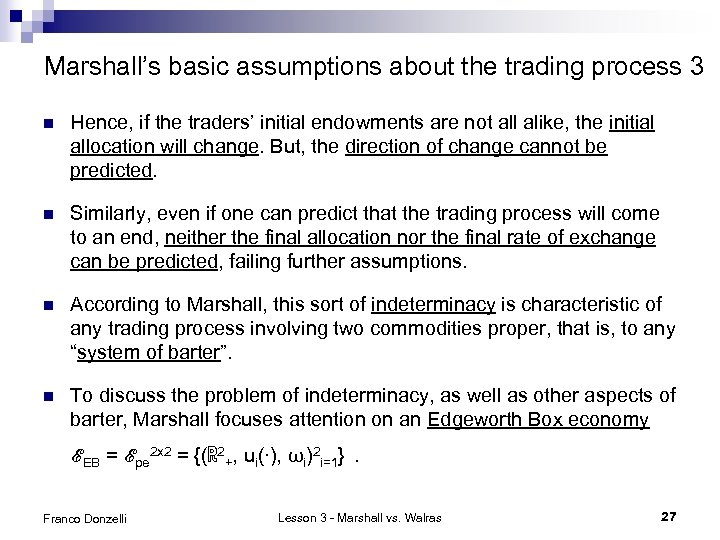Marshall’s basic assumptions about the trading process 3 n Hence, if the traders’ initial endowments are not all alike, the initial allocation will change. But, the direction of change cannot be predicted. n Similarly, even if one can predict that the trading process will come to an end, neither the final allocation nor the final rate of exchange can be predicted, failing further assumptions. n According to Marshall, this sort of indeterminacy is characteristic of any trading process involving two commodities proper, that is, to any “system of barter”. n To discuss the problem of indeterminacy, as well as other aspects of barter, Marshall focuses attention on an Edgeworth Box economy ℰEB = ℰpe 2 x 2 = {(ℝ 2+, ui(∙), ωi)2 i=1}. Franco Donzelli Lesson 3 - Marshall vs. Walras 27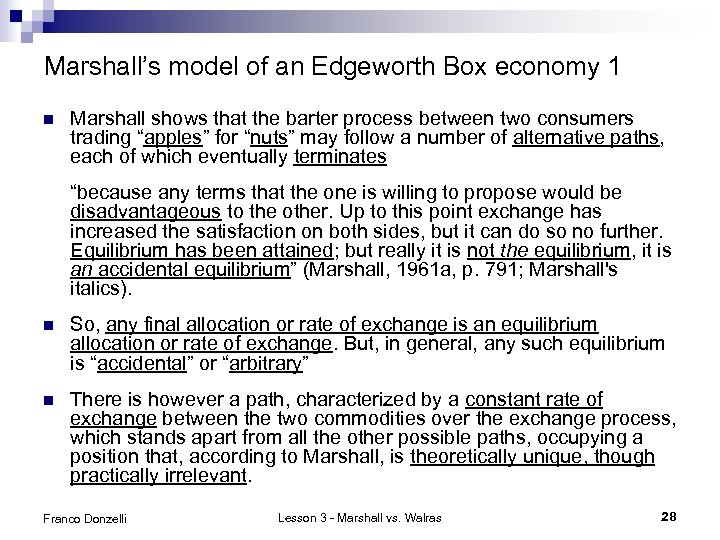Marshall’s model of an Edgeworth Box economy 1 n Marshall shows that the barter process between two consumers trading “apples” for “nuts” may follow a number of alternative paths, each of which eventually terminates “because any terms that the one is willing to propose would be disadvantageous to the other. Up to this point exchange has increased the satisfaction on both sides, but it can do so no further. Equilibrium has been attained; but really it is not the equilibrium, it is an accidental equilibrium” (Marshall, 1961 a, p. 791; Marshall's italics). n So, any final allocation or rate of exchange is an equilibrium allocation or rate of exchange. But, in general, any such equilibrium is “accidental” or “arbitrary” n There is however a path, characterized by a constant rate of exchange between the two commodities over the exchange process, which stands apart from all the other possible paths, occupying a position that, according to Marshall, is theoretically unique, though practically irrelevant. Franco Donzelli Lesson 3 - Marshall vs. Walras 28Marshall’s model of an Edgeworth Box economy 2 n “There is, however, one equilibrium rate of exchange which has some sort of right to be called the true equilibrium rate, because if once hit upon would be adhered to throughout. [. . . ] This is then the true position of equilibrium; but there is no reason to suppose that it will be reached in practice” (Marshall, 1961 a, p. 791) n Let us formalize Marshall’s discussion. Let i =1, 2. Assuming MRS 211(ω11, ω21) ≠ MRS 212(ω12, ω22), let k 12(ω) = min {MRS 211(ω11, ω21), MRS 212(ω12, ω22)} < < max {MRS 211(ω11, ω21), MRS 212(ω12, ω22)} = K 12 (ω) n The Pareto set of ℰEB is the set Franco Donzelli Lesson 3 - Marshall vs. Walras 29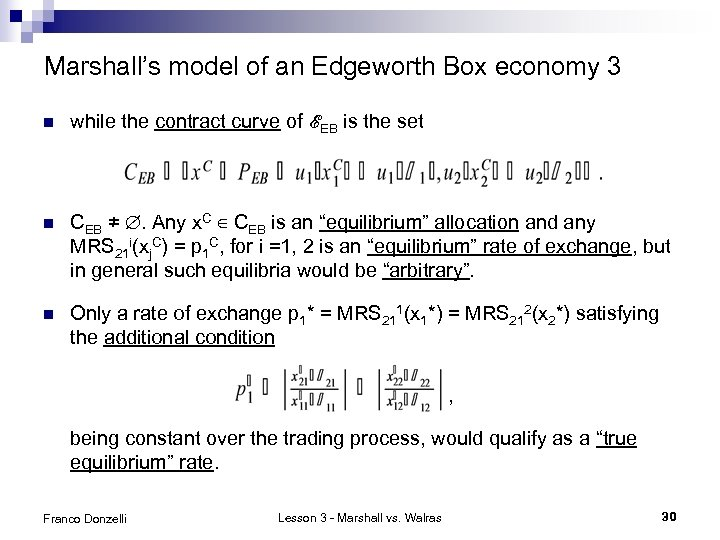Marshall’s model of an Edgeworth Box economy 3 n while the contract curve of ℰEB is the set n CEB ≠ ∅. Any x. C ∈ CEB is an “equilibrium” allocation and any MRS 21 i(xj. C) = p 1 C, for i =1, 2 is an “equilibrium” rate of exchange, but in general such equilibria would be “arbitrary”. n Only a rate of exchange p 1* = MRS 211(x 1*) = MRS 212(x 2*) satisfying the additional condition , being constant over the trading process, would qualify as a “true equilibrium” rate. Franco Donzelli Lesson 3 - Marshall vs. Walras 30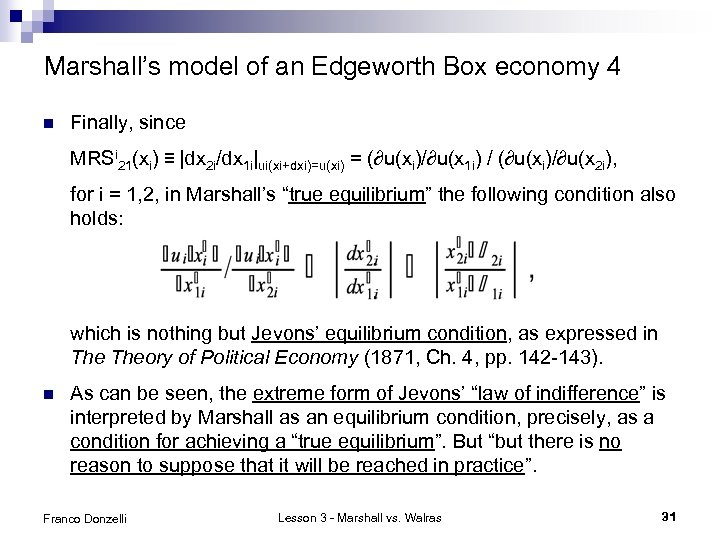Marshall’s model of an Edgeworth Box economy 4 n Finally, since MRSi 21(xi) ≡ |dx 2 i/dx 1 i|ui(xi+dxi)=u(xi) = (∂u(xi)/∂u(x 1 i) / (∂u(xi)/∂u(x 2 i), for i = 1, 2, in Marshall’s “true equilibrium” the following condition also holds: which is nothing but Jevons’ equilibrium condition, as expressed in Theory of Political Economy (1871, Ch. 4, pp. 142 -143). n As can be seen, the extreme form of Jevons’ “law of indifference” is interpreted by Marshall as an equilibrium condition, precisely, as a condition for achieving a “true equilibrium”. But “but there is no reason to suppose that it will be reached in practice”. Franco Donzelli Lesson 3 - Marshall vs. Walras 31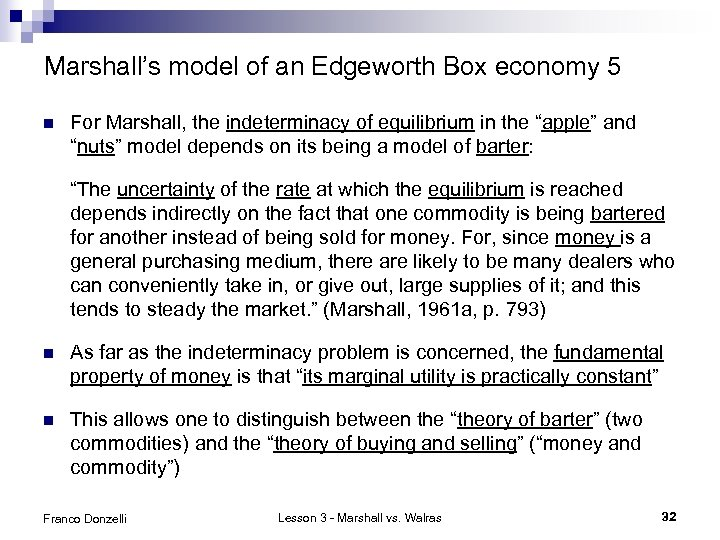Marshall’s model of an Edgeworth Box economy 5 n For Marshall, the indeterminacy of equilibrium in the “apple” and “nuts” model depends on its being a model of barter: “The uncertainty of the rate at which the equilibrium is reached depends indirectly on the fact that one commodity is being bartered for another instead of being sold for money. For, since money is a general purchasing medium, there are likely to be many dealers who can conveniently take in, or give out, large supplies of it; and this tends to steady the market. ” (Marshall, 1961 a, p. 793) n As far as the indeterminacy problem is concerned, the fundamental property of money is that “its marginal utility is practically constant” n This allows one to distinguish between the “theory of barter” (two commodities) and the “theory of buying and selling” (“money and commodity”) Franco Donzelli Lesson 3 - Marshall vs. Walras 32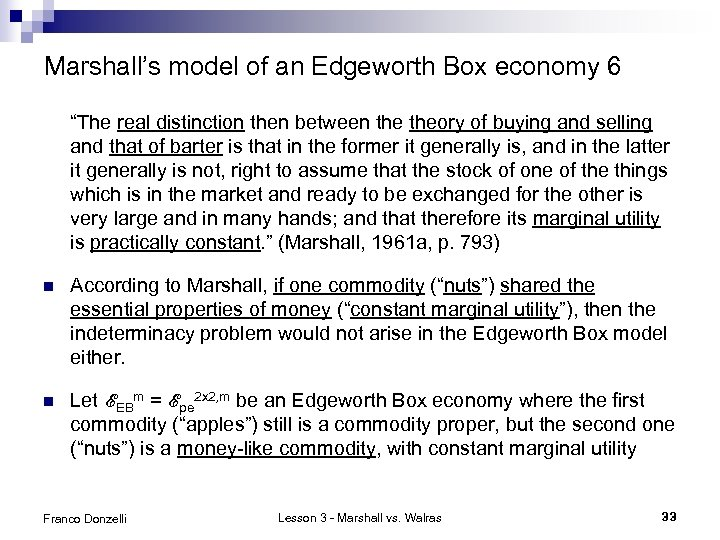Marshall’s model of an Edgeworth Box economy 6 “The real distinction then between theory of buying and selling and that of barter is that in the former it generally is, and in the latter it generally is not, right to assume that the stock of one of the things which is in the market and ready to be exchanged for the other is very large and in many hands; and that therefore its marginal utility is practically constant. ” (Marshall, 1961 a, p. 793) n According to Marshall, if one commodity (“nuts”) shared the essential properties of money (“constant marginal utility”), then the indeterminacy problem would not arise in the Edgeworth Box model either. n Let ℰEBm = ℰpe 2 x 2, m be an Edgeworth Box economy where the first commodity (“apples”) still is a commodity proper, but the second one (“nuts”) is a money-like commodity, with constant marginal utility Franco Donzelli Lesson 3 - Marshall vs. Walras 33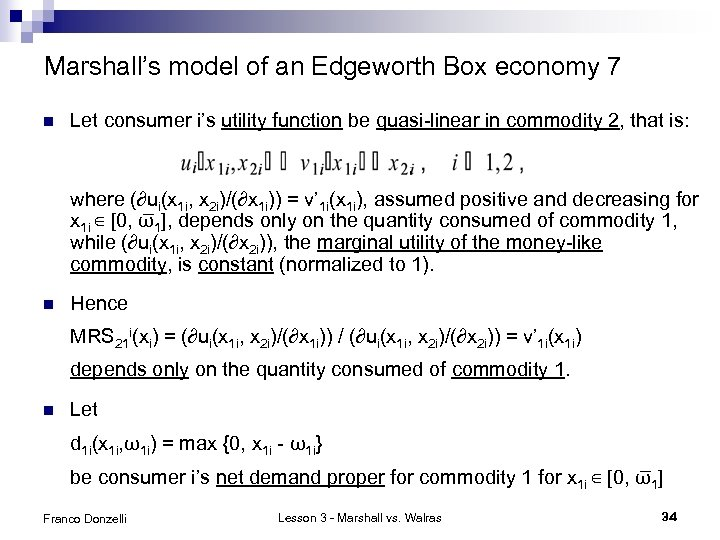Marshall’s model of an Edgeworth Box economy 7 n Let consumer i’s utility function be quasi-linear in commodity 2, that is: where (∂ui(x 1 i, x 2 i)/(∂x 1 i)) = v’ 1 i(x 1 i), assumed positive and decreasing for x 1 i ∈ [0, ω 1], depends only on the quantity consumed of commodity 1, while (∂ui(x 1 i, x 2 i)/(∂x 2 i)), the marginal utility of the money-like commodity, is constant (normalized to 1). n Hence MRS 21 i(xi) = (∂ui(x 1 i, x 2 i)/(∂x 1 i)) / (∂ui(x 1 i, x 2 i)/(∂x 2 i)) = v’ 1 i(x 1 i) depends only on the quantity consumed of commodity 1. n Let d 1 i(x 1 i, ω1 i) = max {0, x 1 i - ω1 i} be consumer i’s net demand proper for commodity 1 for x 1 i ∈ [0, ω 1] Franco Donzelli Lesson 3 - Marshall vs. Walras 34Marshall’s model of an Edgeworth Box economy 8 s 1 i(x 1 i, ω1 i) = |min {0, x 1 i - ω1 i}| be consumer i’s net supply of commodity 1 for x 1 i ∈ [0, ω 1] n If x 1 i > ω1 i, then d 1 i(x 1 i, ω1 i) > 0 and consumer i is a net buyer of commodity 1; hence MRS 21 i(xi) = v’ 1 i(x 1 i) can be interpreted as a buyer’s reservation price, or demand price. n If x 1 i < ω1 i, then s 1 i(x 1 i, ω1 i) > 0 and consumer i is a net seller of commodity 1; hence MRS 21 i(xi) = v’ 1 i(x 1 i) can be interpreted as a seller’s reservation price, or supply price. n Consumer i’s Marshallian inverse supply correspondence of commodity 1, p 1 is: [0, ω1 i] → ℝ+, is defined as follows: p 1 is(s 1 i) = [0, v’ 1 i(x 1 i)] for s 1 i = 0 p 1 is(s 1 i) = v’ 1 i(ω1 i – s 1 i) for s 1 i ∈ (0, ω1 i) (a continuous increasing function) p 1 is(s 1 i) = [v’ 1 i(0), ∞) for s 1 i = ω1 i Franco Donzelli Lesson 3 - Marshall vs. Walras 35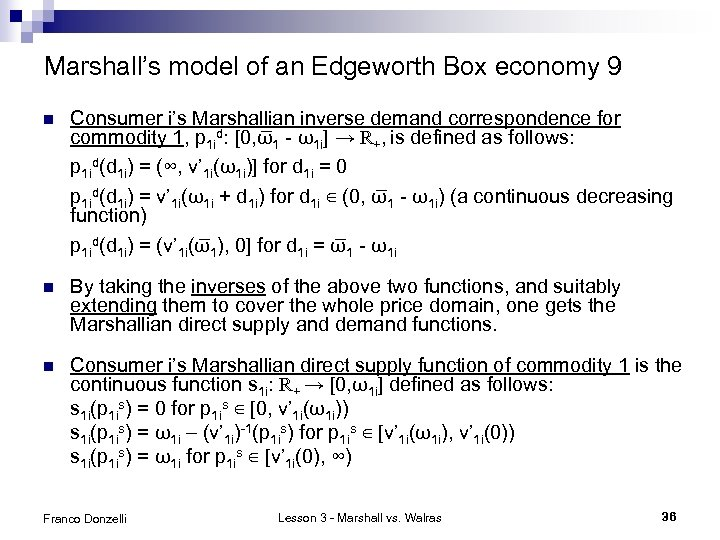Marshall’s model of an Edgeworth Box economy 9 n Consumer i’s Marshallian inverse demand correspondence for commodity 1, p 1 id: [0, ω 1 - ω1 i] → ℝ+, is defined as follows: p 1 id(d 1 i) = (∞, v’ 1 i(ω1 i)] for d 1 i = 0 p 1 id(d 1 i) = v’ 1 i(ω1 i + d 1 i) for d 1 i ∈ (0, ω 1 - ω1 i) (a continuous decreasing function) p 1 id(d 1 i) = (v’ 1 i(ω 1), 0] for d 1 i = ω 1 - ω1 i n By taking the inverses of the above two functions, and suitably extending them to cover the whole price domain, one gets the Marshallian direct supply and demand functions. n Consumer i’s Marshallian direct supply function of commodity 1 is the continuous function s 1 i: ℝ+ → [0, ω1 i] defined as follows: s 1 i(p 1 is) = 0 for p 1 is ∈ [0, v’ 1 i(ω1 i)) s 1 i(p 1 is) = ω1 i – (v’ 1 i)-1(p 1 is) for p 1 is ∈ [v’ 1 i(ω1 i), v’ 1 i(0)) s 1 i(p 1 is) = ω1 i for p 1 is ∈ [v’ 1 i(0), ∞) Franco Donzelli Lesson 3 - Marshall vs. Walras 36Marshall’s model of an Edgeworth Box economy 10 n Consumer i’s Marshallian direct demand function for commodity 1 is the continuous function d 1 i: ℝ+ → [0, ω 1 - ω1 i] defined as follows: d 1 i(p 1 id) = 0 for p 1 id ∈ (∞, v’ 1 i(ω1 i)] d 1 i(p 1 is) = (v’ 1 i)-1(p 1 id) - ω1 i for p 1 id ∈ (v’ 1 i(ω1 i), v’ 1 i(ω 1)] d 1 i(p 1 id) = ω 1 - ω1 i for p 1 id ∈ (v’ 1 i(ω 1), 0] n Let p 1 mind = mini {v’ 1 i(ω 1)} and p 1 maxd = maxi {v’ 1 i(ω1 i)}; let p 1 mins = mini {v’ 1 i(ω1 i)} and p 1 maxs = maxi {v’ 1 i(0)}. n If the consumers’ tastes are not identical, then p 1 maxd > p 1 mins n Let d 1(p 1 d) = ∑i d 1 i(p 1 id), for p 1 d = p 1 id, for i = 1, 2 and p 1 d ∈ [0, ∞); let s 1(p 1 s) = ∑i s 1 i(p 1 is), for p 1 s = p 1 is, for i = 1, 2 and p 1 s ∈ [0, ∞). Franco Donzelli Lesson 3 - Marshall vs. Walras 37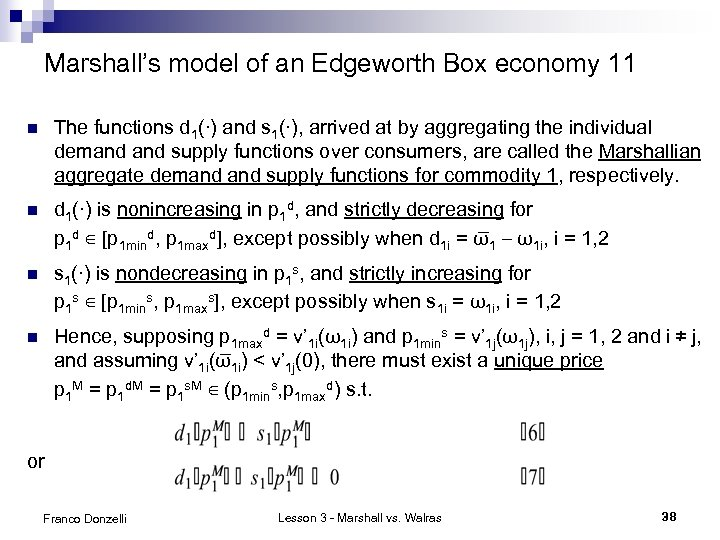Marshall’s model of an Edgeworth Box economy 11 n The functions d 1(∙) and s 1(∙), arrived at by aggregating the individual demand supply functions over consumers, are called the Marshallian aggregate demand supply functions for commodity 1, respectively. n d 1(∙) is nonincreasing in p 1 d, and strictly decreasing for p 1 d ∈ [p 1 mind, p 1 maxd], except possibly when d 1 i = ω 1 – ω1 i, i = 1, 2 n s 1(∙) is nondecreasing in p 1 s, and strictly increasing for p 1 s ∈ [p 1 mins, p 1 maxs], except possibly when s 1 i = ω1 i, i = 1, 2 n Hence, supposing p 1 maxd = v’ 1 i(ω1 i) and p 1 mins = v’ 1 j(ω1 j), i, j = 1, 2 and i ≠ j, and assuming v’ 1 i(ω 1 i) < v’ 1 j(0), there must exist a unique price p 1 M = p 1 d. M = p 1 s. M ∈ (p 1 mins, p 1 maxd) s. t. or Franco Donzelli Lesson 3 - Marshall vs. Walras 38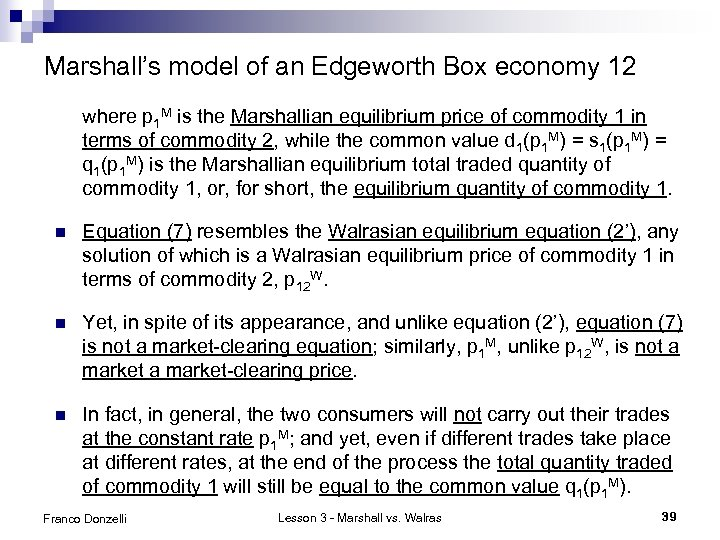Marshall’s model of an Edgeworth Box economy 12 where p 1 M is the Marshallian equilibrium price of commodity 1 in terms of commodity 2, while the common value d 1(p 1 M) = s 1(p 1 M) = q 1(p 1 M) is the Marshallian equilibrium total traded quantity of commodity 1, or, for short, the equilibrium quantity of commodity 1. n Equation (7) resembles the Walrasian equilibrium equation (2’), any solution of which is a Walrasian equilibrium price of commodity 1 in terms of commodity 2, p 12 W. n Yet, in spite of its appearance, and unlike equation (2’), equation (7) is not a market-clearing equation; similarly, p 1 M, unlike p 12 W, is not a market-clearing price. n In fact, in general, the two consumers will not carry out their trades at the constant rate p 1 M; and yet, even if different trades take place at different rates, at the end of the process the total quantity traded of commodity 1 will still be equal to the common value q 1(p 1 M). Franco Donzelli Lesson 3 - Marshall vs. Walras 39Marshall’s model of an Edgeworth Box economy 13 n An illustration: q Assumption 1. (Utility functions quadratic in commodity 1 and quasi-linear in commodity 2) ui(x 1 i, x 2 i) = v 1 i(x 1 i) + v 2 i(x 2 i) = ai(x 1 i - ω1 i) - ½bi(x 1 i - ω1 i)2 + x 2 i, i = 1, 2. Hence: MRS 21 i(xi) = v’ 1 i(x 1 i) = ai - bi(x 1 i - ω1 i) , i = 1, 2. q Assumption 2. K 12(ω) = MRS 211(ω1) = a 1 > a 2 = MRS 212(ω2) = k 12(ω) q Assumption 3. ω11 < ω12 ; v’ 11(ω 1) < v’ 12(0) Equilibrium: p 11 d(d 11) = p 11 s(s 12) and d 11 = s 12. Hence: p 1 M = (a 1 b 2 + a 2 b 1) / (b 1 + b 2) ; q 1 M = (a 1 - a 2) / (b 1 + b 2) Franco Donzelli Lesson 3 - Marshall vs. Walras 40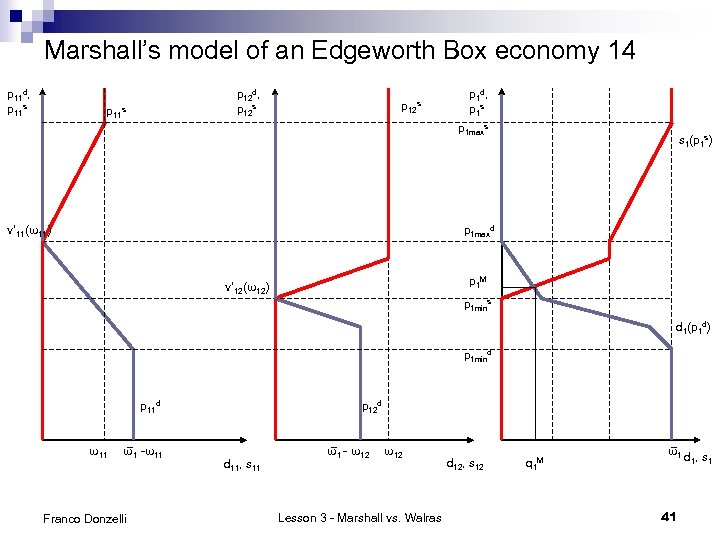Marshall’s model of an Edgeworth Box economy 14 p 11 d, p 11 s p 12 d, p 12 s p 11 s p 12 s p 1 d, p 1 s p 1 maxs v’ 11(ω11) s 1(p 1 s) p 1 maxd p 1 M v’ 12(ω12) p 1 mins d 1(p 1 d) p 1 mind p 11 d ω11 ω 1 -ω11 Franco Donzelli p 12 d d 11, s 11 ω 1 - ω12 Lesson 3 - Marshall vs. Walras d 12, s 12 q 1 M ω 1 d , s 1 1 41Marshall’s model of an Edgeworth Box economy 15 n When the two consumers have already cumulatively traded a quantity q 1 of commodity 1, such that q 1 ∈ [0, q 1(p 1 M)), there still exists a positive difference between the demand the supply price of commodity 1 corresponding to q 1, that is p 1 d(q 1) - p 1 s(q 1) > 0. n Hence there still is room for a weakly advantageous marginal trade between the two consumers, at any rate of exchange p 1 ∈ [p 1 d(q 1), p 1 s(q 1)] n The rate of exchange p 1 M ought to be interpreted as the final rate to which the sequence of the rates at which the consumers have traded during the trading process necessarily converges, along a path which may exhibit no regularity other than the stated convergence. n The total quantity of commodity 1 traded by the traders, q 1(p 1 M)), ought instead to be interpreted as the quantity of commodity 1 to which the monotonically increasing sequence of the quantities cumulatively traded by the consumers during the exchange process necessarily converges. Franco Donzelli Lesson 3 - Marshall vs. Walras 42Marshall’s model of an Edgeworth Box economy 14 n The total quantity of the money-like commodity 2 cumulatively traded by the consumers at the end of the trading process remains undetermined, its final value being however necessarily confined to the interval , where i, j = 1, 2, i ≠ j; i, j are s. t. v’ 1 i(ω1 i) = p 1 mins and v’ 1 j(ω1 j) = p 1 maxd. n Hence in Marshall’s model there exists no counterpart of equation (2’’) in Walras’s model, where it provides the market-clearing condition for commodity 2. n Further, in Marshall’s model there is nothing comparable to Walras’ Law, even if, due to the bilateral character of any exchange, the total value of sales must always equal that of purchases for each consumer, hence for the whole economy. Franco Donzelli Lesson 3 - Marshall vs. Walras 43Marshall’s “temporary equilibrium” model 1 n Marshall's "temporary equilibrium" model actually consists in a limited extension of his Edgeworth Box model with a money-like commodity to a pure-exchange, two-commodity economy with an arbitrary finite number of traders, that is, an economy ℰpe 2 x. I, m = {(ℝ 2+, ui(∙), ωi)Ii=1} with I >2, where commodity 1 is a consumers' good, commodity 2 is money, and the marginal utility of commodity 2 is assumed to be constant. n An ambiguity of the model: ¨ ¨ n formally: an entire economy → general equilibrium analysis substantially: a single market → partial equilibrium analysis Effects on the possibility of formalizing Marshall’s empirical justifications for assuming the “marginal utility of money” to be “constant”: ¨ money should be “in large supply and general use” (possible) ¨ the expenditure on the good for which money is traded should represent “a small part of [each trader’s] resources” (meaningless) Franco Donzelli Lesson 3 - Marshall vs. Walras 44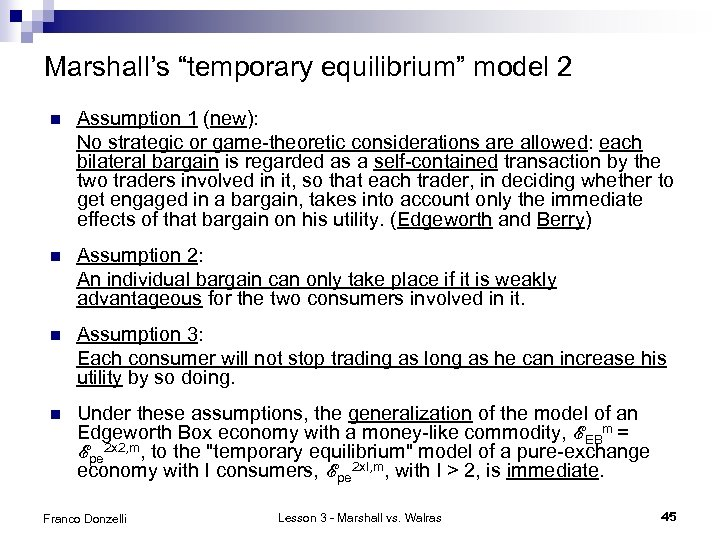Marshall’s “temporary equilibrium” model 2 n Assumption 1 (new): No strategic or game-theoretic considerations are allowed: each bilateral bargain is regarded as a self-contained transaction by the two traders involved in it, so that each trader, in deciding whether to get engaged in a bargain, takes into account only the immediate effects of that bargain on his utility. (Edgeworth and Berry) n Assumption 2: An individual bargain can only take place if it is weakly advantageous for the two consumers involved in it. n Assumption 3: Each consumer will not stop trading as long as he can increase his utility by so doing. n Under these assumptions, the generalization of the model of an Edgeworth Box economy with a money-like commodity, ℰEBm = ℰpe 2 x 2, m, to the "temporary equilibrium" model of a pure-exchange economy with I consumers, ℰpe 2 x. I, m, with I > 2, is immediate. Franco Donzelli Lesson 3 - Marshall vs. Walras 45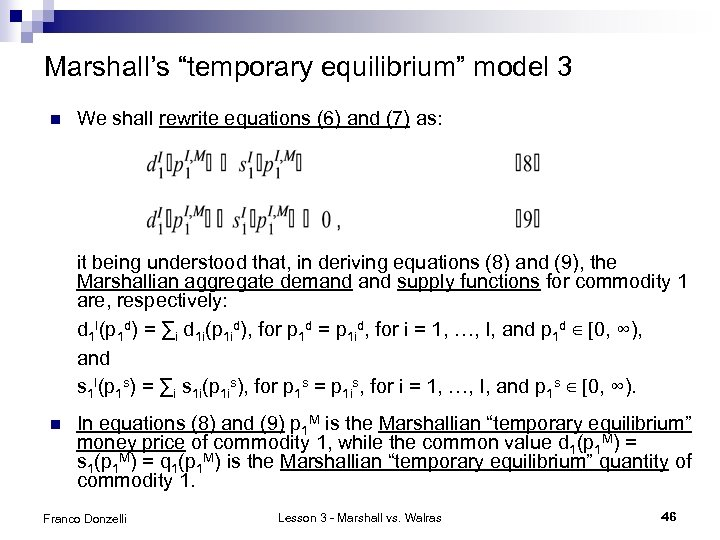Marshall’s “temporary equilibrium” model 3 n We shall rewrite equations (6) and (7) as: it being understood that, in deriving equations (8) and (9), the Marshallian aggregate demand supply functions for commodity 1 are, respectively: d 1 I(p 1 d) = ∑i d 1 i(p 1 id), for p 1 d = p 1 id, for i = 1, …, I, and p 1 d ∈ [0, ∞), and s 1 I(p 1 s) = ∑i s 1 i(p 1 is), for p 1 s = p 1 is, for i = 1, …, I, and p 1 s ∈ [0, ∞). n In equations (8) and (9) p 1 M is the Marshallian “temporary equilibrium” money price of commodity 1, while the common value d 1(p 1 M) = s 1(p 1 M) = q 1(p 1 M) is the Marshallian “temporary equilibrium” quantity of commodity 1. Franco Donzelli Lesson 3 - Marshall vs. Walras 46Marshall’s “temporary equilibrium” model 4 n Marshall’s interpretation of equations (8) and (9) is essentially the same as that of equations (6) and (7). Yet Marshall’s claims are not entirely justified. n Marshall’s “temporary equilibrium” model is developed by means of an example, referring to “a corn market in a country town”, where “corn” is traded for “money”. The illustration is based on the “facts” summarized by the following “table” (Marshall, 1961 a, pp. 332 -333): Franco Donzelli Lesson 3 - Marshall vs. Walras 47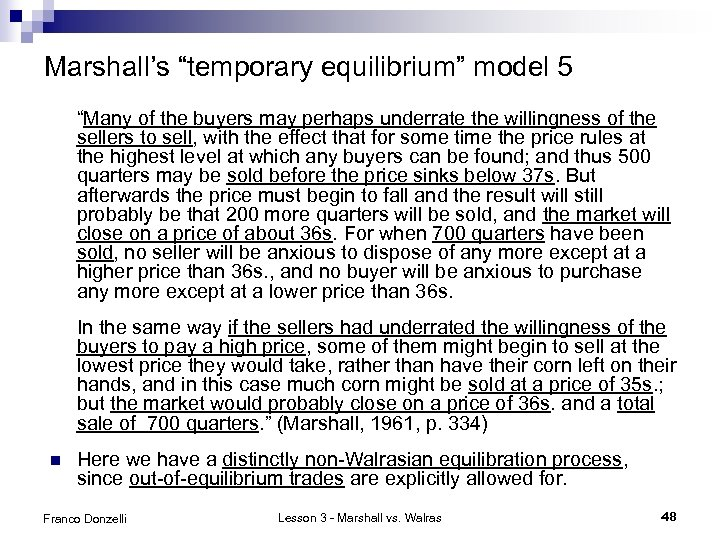Marshall’s “temporary equilibrium” model 5 “Many of the buyers may perhaps underrate the willingness of the sellers to sell, with the effect that for some time the price rules at the highest level at which any buyers can be found; and thus 500 quarters may be sold before the price sinks below 37 s. But afterwards the price must begin to fall and the result will still probably be that 200 more quarters will be sold, and the market will close on a price of about 36 s. For when 700 quarters have been sold, no seller will be anxious to dispose of any more except at a higher price than 36 s. , and no buyer will be anxious to purchase any more except at a lower price than 36 s. In the same way if the sellers had underrated the willingness of the buyers to pay a high price, some of them might begin to sell at the lowest price they would take, rather than have their corn left on their hands, and in this case much corn might be sold at a price of 35 s. ; but the market would probably close on a price of 36 s. and a total sale of 700 quarters. ” (Marshall, 1961, p. 334) n Here we have a distinctly non-Walrasian equilibration process, since out-of-equilibrium trades are explicitly allowed for. Franco Donzelli Lesson 3 - Marshall vs. Walras 48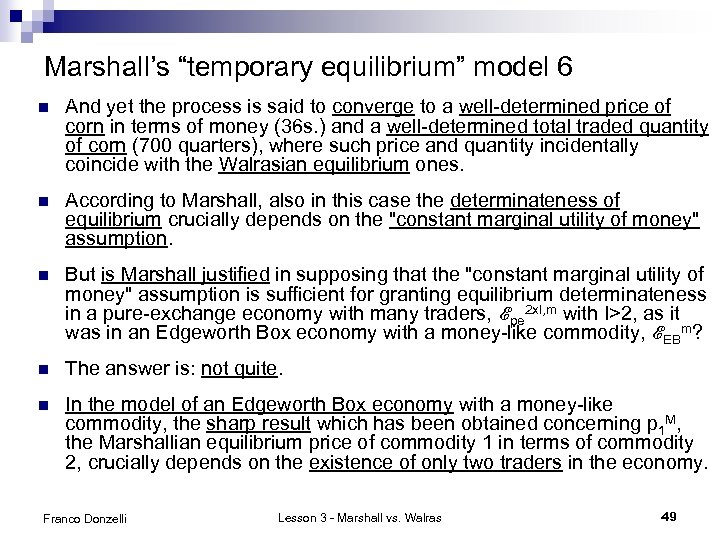Marshall’s “temporary equilibrium” model 6 n And yet the process is said to converge to a well-determined price of corn in terms of money (36 s. ) and a well-determined total traded quantity of corn (700 quarters), where such price and quantity incidentally coincide with the Walrasian equilibrium ones. n According to Marshall, also in this case the determinateness of equilibrium crucially depends on the "constant marginal utility of money" assumption. n But is Marshall justified in supposing that the "constant marginal utility of money" assumption is sufficient for granting equilibrium determinateness in a pure-exchange economy with many traders, ℰpe 2 x. I, m with I>2, as it was in an Edgeworth Box economy with a money-like commodity, ℰEBm? n The answer is: not quite. n In the model of an Edgeworth Box economy with a money-like commodity, the sharp result which has been obtained concerning p 1 M, the Marshallian equilibrium price of commodity 1 in terms of commodity 2, crucially depends on the existence of only two traders in the economy. Franco Donzelli Lesson 3 - Marshall vs. Walras 49Marshall’s “temporary equilibrium” model 7 n With only two traders, the marginal rate of exchange at which the last marginal trade occurs necessarily coincides with both the marginal demand price of the only marginal buyer, p 1 d(q 1(p 1 M)), and the marginal supply price of the only marginal seller, p 1 s(q 1(p 1 M)). n Hence, assuming uniqueness of the equilibrium price, it also necessarily coincides with p 1 M, which can therefore be legitimately interpreted as the final rate to which the sequence of the rates at which the traders have traded during the exchange process necessarily converges. n But in Marshall's "temporary equilibrium" model there are more than two traders in the economy: hence, in general, not only there may exist more than one marginal buyer or seller, but also there may be some buyers or sellers that are not marginal. n Due to this, in Marshall's "temporary equilibrium" model the total quantity of commodity 1 traded in the market still converges to the Marshallian "temporary equilibrium" quantity, q 1(p 1 M), but the sequence of the money prices of commodity 1 at which the traders buy and sell that commodity during the trading process no longer necessarily converges to the Marshallian "temporary equilibrium" price, p 1 I, M: the outcome depends on the order of the matchings. Franco Donzelli Lesson 3 - Marshall vs. Walras 50Marshall’s pure-exchange models: limitations and extensions 1 n Marshall’s Edgeworth Box model is propedeutical to his “temporary equilibrium” model, which is in turn propedeutical to Marshall’s normal equilibrium models. n But, even if propedeutical, Marshall’s pure-exchange models still play a fundamental role in the overall structure of Marshall’s thought. n In his pure-exchange, two-commodity models Marshall wants to show an equilibrium comes to be established as the final outcome of a realistic process of exchange in "real" time, where trades can actually take place at out-of-equilibrium rates of exchange or prices. n This program inevitably raises the issue of equilibrium determinacy. n Marshall's solution consists in imposing some related restrictions on the traders' utility functions, which are assumed to be quasi-linear in one of the two commodities, and the nature of the commodities themselves, one of which is interpreted as a money-like commodity or money tout court. Franco Donzelli Lesson 3 - Marshall vs. Walras 51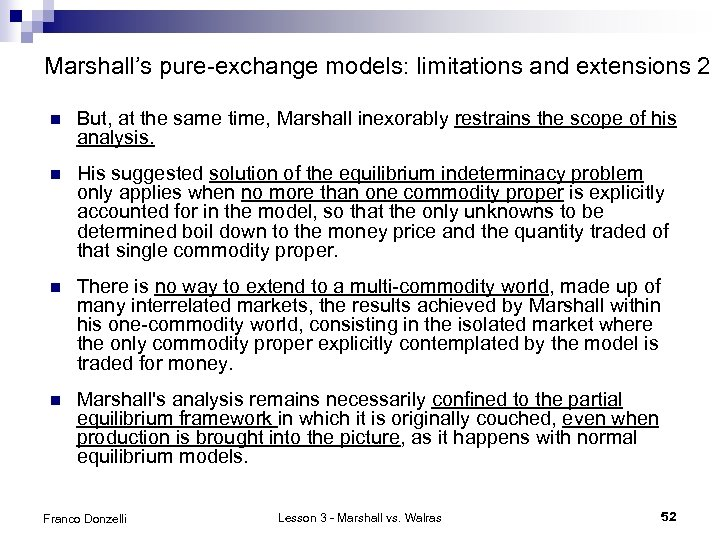Marshall’s pure-exchange models: limitations and extensions 2 n But, at the same time, Marshall inexorably restrains the scope of his analysis. n His suggested solution of the equilibrium indeterminacy problem only applies when no more than one commodity proper is explicitly accounted for in the model, so that the only unknowns to be determined boil down to the money price and the quantity traded of that single commodity proper. n There is no way to extend to a multi-commodity world, made up of many interrelated markets, the results achieved by Marshall within his one-commodity world, consisting in the isolated market where the only commodity proper explicitly contemplated by the model is traded for money. n Marshall's analysis remains necessarily confined to the partial equilibrium framework in which it is originally couched, even when production is brought into the picture, as it happens with normal equilibrium models. Franco Donzelli Lesson 3 - Marshall vs. Walras 52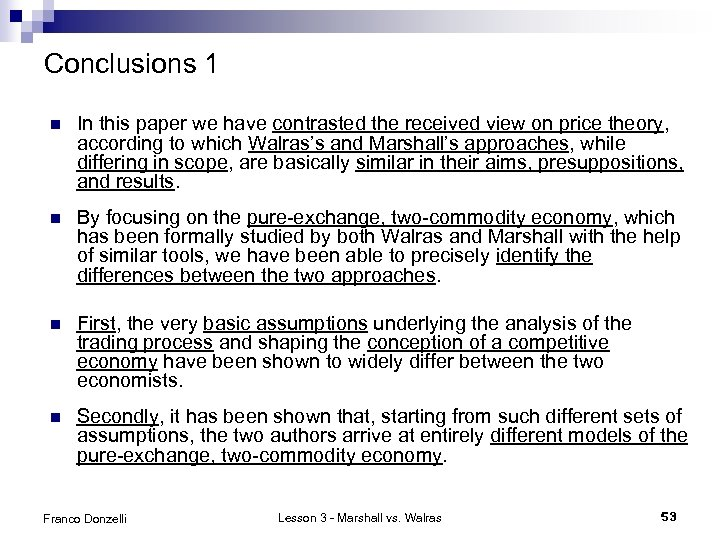Conclusions 1 n In this paper we have contrasted the received view on price theory, according to which Walras’s and Marshall’s approaches, while differing in scope, are basically similar in their aims, presuppositions, and results. n By focusing on the pure-exchange, two-commodity economy, which has been formally studied by both Walras and Marshall with the help of similar tools, we have been able to precisely identify the differences between the two approaches. n First, the very basic assumptions underlying the analysis of the trading process and shaping the conception of a competitive economy have been shown to widely differ between the two economists. n Secondly, it has been shown that, starting from such different sets of assumptions, the two authors arrive at entirely different models of the pure-exchange, two-commodity economy. Franco Donzelli Lesson 3 - Marshall vs. Walras 53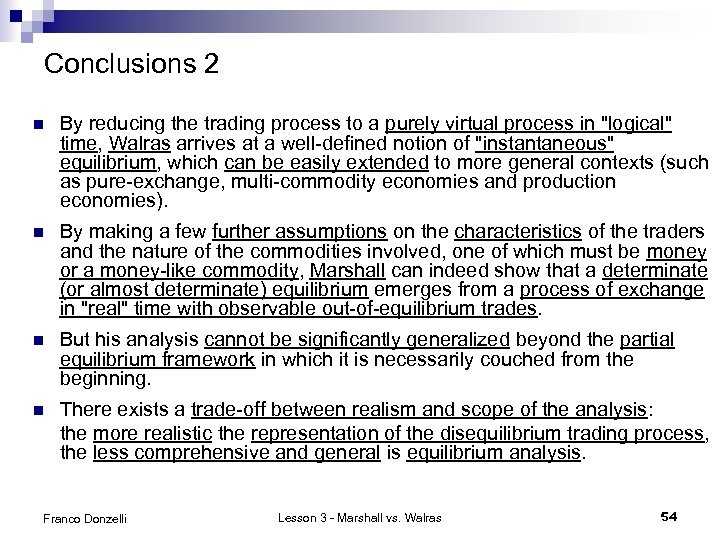Conclusions 2 n By reducing the trading process to a purely virtual process in "logical" time, Walras arrives at a well-defined notion of "instantaneous" equilibrium, which can be easily extended to more general contexts (such as pure-exchange, multi-commodity economies and production economies). n By making a few further assumptions on the characteristics of the traders and the nature of the commodities involved, one of which must be money or a money-like commodity, Marshall can indeed show that a determinate (or almost determinate) equilibrium emerges from a process of exchange in "real" time with observable out-of-equilibrium trades. n But his analysis cannot be significantly generalized beyond the partial equilibrium framework in which it is necessarily couched from the beginning. n There exists a trade-off between realism and scope of the analysis: the more realistic the representation of the disequilibrium trading process, the less comprehensive and general is equilibrium analysis. Franco Donzelli Lesson 3 - Marshall vs. Walras 54